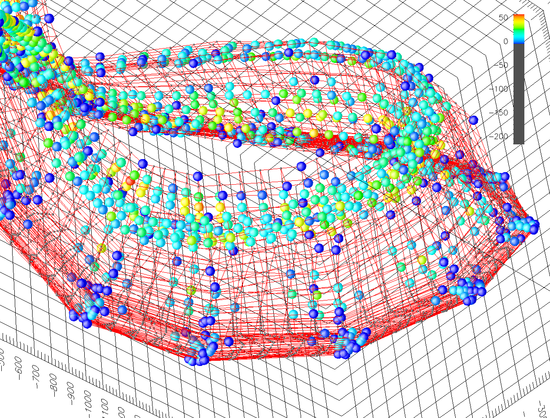# Research Institute of Visual Computing

Select a project of interest using the advanced search:

# Image manifolds as a way of representing the appearance of objectsImages of objects (such as a rotating teapot, views from a camera mounted on a robot, an actor illuminated from different positions on a hemisphere, etc.) which vary between them by some controlled parameter tend not to be randomly located in the space of all possible x × y pixel images, where each of the c colour components of each pixel forms one coordinate of a x  × y × cdimensional position vector. This high-dimensional space is often referred to as image space.

Under certain circumstances these families of images can be approximated as n-manifolds, where n is the number of independent variables controlling the image transformations, which we call image manifolds. This mapping between the high-dimensional image space and a lower dimensional sub-space has been particularly useful in the area of vision, however developing efficient in-memory representations of image manifolds has proven hard, and many researchers have used techniques like PCA to simplify the representations. This is acceptable if the intended use is recognition of images. However for the purpose of synthesising new images it proves to lose too many of the details that make images appear realistic to a human observer.

We develop existing surface representation techniques (typically PDE and NURBS) to model these structures in image space directly, thereby exactly representing all of the images that make up the manifold. Such models then allow the exact reconstruction of interpolating images by simply evaluating the manifold at the desired location in parameter space.

The Figure shows an example of a manifold built using PDE surfaces to model a rotating and translating teapot (only the three major components of a PCA projection are shown). Dark blue spheres correspond to the images used to construct the manifold. Lighter spheres correspond to images from the manifold that are better than their linearly interpolated equivalent. Very small grey spheres correspond to images where the linear interpolation is better than the image created from the manifold.